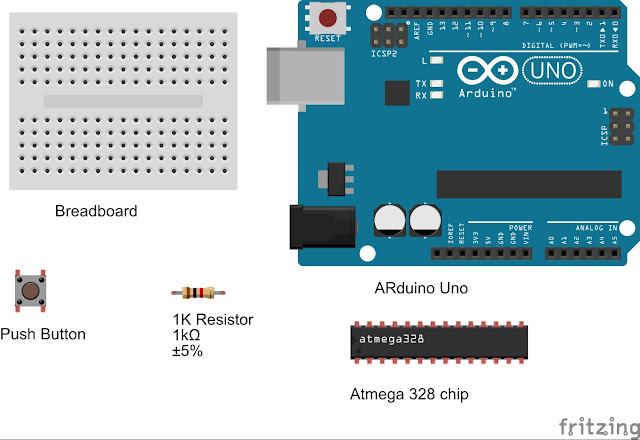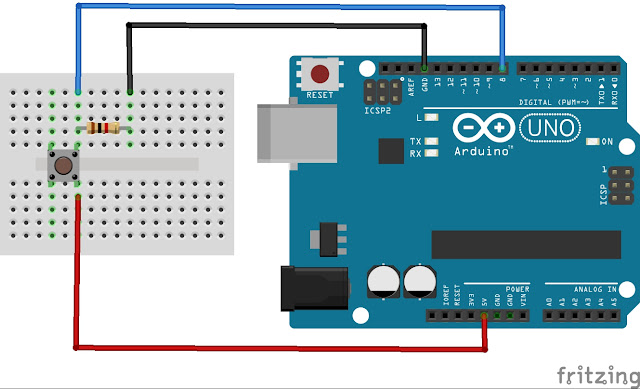# Arduino Tutorial - ButtonsThis is a very important tutorial for beginners as you can do major projects using this theory. Only thing is that you have to think beyond the normal.

I will explain one by one. first of all shall we collect the necessary parts for this project. You may need following parts.Let's do the wiring.Lets start coding.

I have assiged here int variable for 13 pin of arduino (on board led) and I call that pin as led.Then i make the variable

int led = 13;

another variable for the "push button" . I assiged again int variable to Pin number 8 and i call it as button.

int button = 8;

now I want to light the LED therefore I set the pin number 13 (led) as a output.

pinMode(led,OUTPUT);

and also we get the signal from Button to arduino, we set that pin 8 as input.

pinMode(button,INPUT);

As i have connected GROUND wire to input pin 8 (button) its current always 0. If is push the button , 5v pin connect with the pin 8, then the current of pin 8 become 1. ( 1 = 5v). In arduino it 5v may be called as "1" or "HIGH" and Ground volt may be called as "0" or "LOW".

Now i want to get the value of pin 8. so i use the following code.

digitalRead(button); if the button is pressed, this will be "1" else "0"

Now you can understand what shall we do. we use "if" command.

digitalWrite(led,HIGH); // we use digitalWrite command to get out put pin voltage 5v or 0.
delay(300);  }// delay(1000) = 1 second. here the programme will stop a while.
else{
digitalWrite(led,LOW);} // off the led

Full code is like thisYou can see now if you press the button, LED ON and release the button LED off.
If you press and hold the button LED continuously on and off

another issue is there. IF you press the button LED on and wait a while due to the delay function. Until the delay time is over the programme do not run the codes. If we have added another code after this button code we can not run both codes simultaneously.

Due to those matters we have to do some changes to the code.

``````/*

/**
* Arduino Button
*/

int led = 13;
int button = 8;
int Bstatus=LOW;
int current;
int newtime;
int x = LOW;
int i =  0;

void setup() {
// put your setup code here, to run once:
pinMode(led,OUTPUT);
pinMode(button,INPUT);
//Serial.begin(9600);
}

void loop() {
// put your main code here, to run repeatedly:

//for this purpose we can use millis command

current = millis();

if (digitalRead(button)==HIGH){ // or you can use as 1
//Serial.println("Button pressed");
//delay(1000); //
if ((current - newtime) > 200){ // change 200 then you can control the speed.
newtime = current ;
i = i+1;

if (i > 1){i=0;}

if (i == 0) {
x = LOW;
}
else{ x = HIGH;}

}
//newtime = current ; //if you want to on/off when press and hold the button then
}

digitalWrite(led,x);
//Serial.println("Button Button released");

}```

```
`Watch the video for more details`
```
```